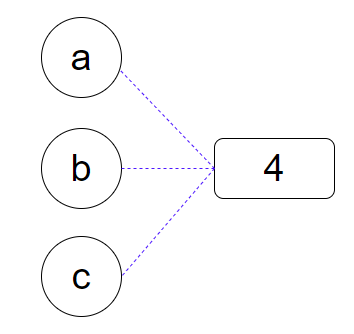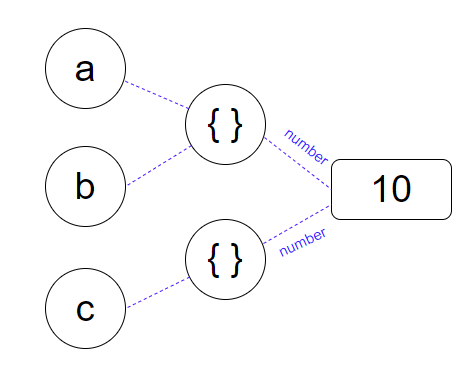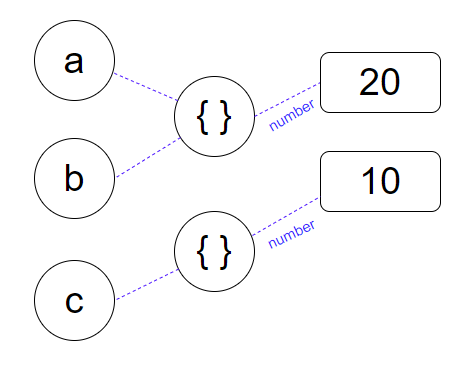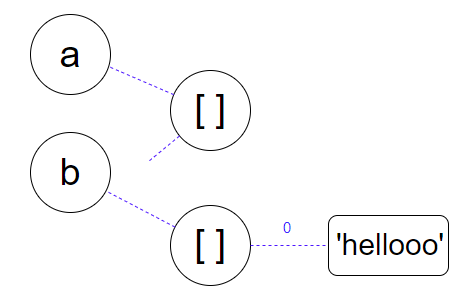# JavaScript: Data Type & Variable Assignment

## JavaScript 資料型態

JavaScript 資料型態可以分為原始型態（Primitive type）和物件型態（Object type）：

• null (null)
• undefined (undefined)
• string ("hello")
• number (5487)
• boolean (true, false)
• symbol (Sym)
• bigints (8n)

• object (object, array, function, date)

## 資料型態的檢查

### Typeof

typeof 可以用來檢查資料的型態，但要注意 typeof null 回傳的是 object，這是 JavaScript 的歷史 bug。

if (typeof a !== 'undefined') {
console.log(a);
}


### Array.isArray()

Array.isArray() 可以用來檢查是不是陣列：

var a = [1, 2, 3, 4];
Array.isArray(a);
// true


### Object.prototype.toString.call()

Object.prototype.toString.call() 更精確地檢查資料的型態：

var a = [1, 2, 3, 4];
Object.prototype.toString.call(a);
// "[object Array]"


### 關於 == 與 === 的迷思

var a = 1;
var b = '1';
console.log(a == b); // true
console.log(a === b); // false


"== is not about comparisons with unknown types, == is about comparisons with known type(s) optionally where conversions are helpful."
-- Kyle Simpson

### NaN

var a = Number('hello')

console.log(type of a)
// NaN

console.log(a === a)
// false


## 變數的宣告與賦值

var a;
var b = 1;
b = 2;


"Variables are not values.
Variables point to values."
-- Dan Abramov

undeclare ≠ undefined

var b;
console.log(a) // Uncaught ReferenceError: b is not define
console.log(b) // undefined


## 原始型態（Primitive type）

### Immutable

let a = 4;
let b = a;
let c = 2 + 2;var a = 10;
var b = a;
b = 200;
console.log(a, b);
// 10 200


### Pass by value

function test(x) {
x = x * 2;
}

var a = 10;
test(a);
console.log(a); // 10


## 物件型態（Object type）

### Mutable

for (var i = 0; i < 3; i++) {
console.log(2);
}


for (var i = 0; i < 3; i++) {
console.log({});
}


var a = {}
var b = a;
var c = {}

console.log(a, b, c);
// {} {} {}

1. 創造一個新的物件 { }，讓 a 指向這個物件
2. 讓 b 指向 a 所指向的物件
3. 創造一個新的物件 { }，讓 a 指向這個物件

### Property

var a = {
number: 10;
}

var b = a;
var c = {
number: 10;
}

console.log(a, b);
// { number: 10 } { number: 10 } { number: 10 }### 改變物件 Property 的指向

var a = {
number: 10;
}

var b = obj;
obj2.number = 20;

console.log(a, b, c);
// { number: 20 } { number: 20 } { number: 10 }

console.log(a === b);var a = [];
var b = a;
a = ['hellooo'];
console.log(a, b);

// [] ['arr2']

1. 把變數 a 賦值為 [ ]，創造一個 [ ]，讓變數 a 指向 [ ]，
2. 把變數 b 賦值為 a ，意思讓 b 去指向 a 所指向的那個 [ ]。
3. 把 b 重新賦值為 ['hellooo'] ，創造一個新的 [ ]，讓 arr 2 去指向它，而這個陣列裡，把第一個參數當成一條線，去指向獨一無二的 hellooo。### Property 永遠指向值

let person1 = {
name: 'Nicolas',
home: { city: 'Taipei' }
};

let person2 = {
name: 'Matt',
home: person1.home
};


### let & const

ES6 的 const 宣告之後之後就不能再重新賦值了，也就是不能重新指向別的值，所以一開始宣告的時候就要賦值。而 let 可以被重新賦值，但又不會像 var 一樣汙染到外部的變數。

const a = {
number: 1
}

a = {
number: 2
}


const obj = {
number: 1
}

obj.number = 2


### Pass by sharing

var coin1 = { value: 10 };

function changeValue(obj) {
obj = { value: 123 };
}

changeValue(coin1);
console.log(coin1);   // 此時 coin1 仍是 { value: 10 }


## 總結

### 參考資料（也推薦閱讀）

Dan Abramov 的 Just JavaScript

MDN web docs - The arguments object